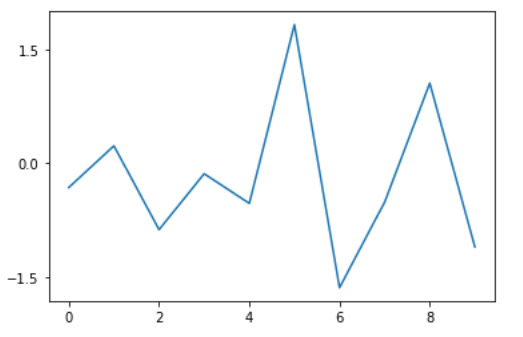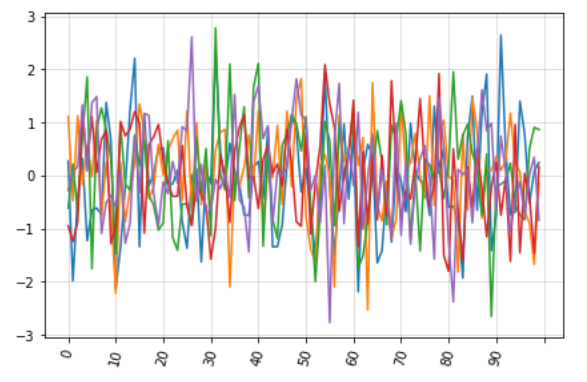# Matplotlib.ticker.MaxNLocator Class in Python

Matplotlib is an amazing visualization library in Python for 2D plots of arrays. Matplotlib is a multi-platform data visualization library built on NumPy arrays and designed to work with the broader SciPy stack.

## matplotlib.ticker.MaxNLocator

The `matplotlib.ticker.MaxNLocator` class is used to select no more than N intervals at nice locations. It is a subclass of `matplotlib.ticker.Locator`.

Syntax: class matplotlib.ticker.MaxNLocator(*args, **kwargs)

Parameter:

• nbins: It is either an integer or ‘auto’, where the integer value represents the maximum number of intervals; one less than max number of ticks. The number of bins gets automatically determined on the basis of the length of the axis.It is an optional argument and has a default value of 10.
• steps: It is an optional parameter representing a nice number sequence that starts from 1 and ends with 10.
• integer: It is an optional boolean value. If set True, the ticks accepts only integer values, provided at least min_n_ticks integers are within the view limits.
• symmetric: It is an optional value. If set to True, auto-scaling will result in a range symmetric about zero.
• prune: It is an optional parameter that accepts either of the four values: {‘lower’, ‘upper’, ‘both’, None}. By default it is None.

Methods of the class:

• set_params(self, **kwargs): It sets parameters for the locator.
• tick_values(self, vmin, vmax): It returns the values of the located ticks given vmin and vmax.
• view_limits(self, dmin, dmax): It is used to select a scale for the range from vmin to vmax.

Example 1:

 `import` `matplotlib.pyplot as plt ` `from` `matplotlib ``import` `ticker ` `import` `numpy as np ` ` `  ` `  `N ``=` `10` `x ``=` `np.arange(N) ` `y ``=` `np.random.randn(N) ` ` `  `fig ``=` `plt.figure() ` `ax ``=` `fig.add_subplot(``111``) ` `ax.plot(x, y) ` ` `  `# Create your ticker object with M ticks ` `M ``=` `3` `yticks ``=` `ticker.MaxNLocator(M) ` ` `  `# Set the yaxis major locator using ` `# your ticker object.  ` `ax.yaxis.set_major_locator(yticks) ` ` `  `plt.show() `

Output:Example 2:

 `import` `matplotlib.pyplot as plt ` `from` `matplotlib.ticker ``import` `MaxNLocator, IndexFormatter ` ` `  ` `  `ax ``=` `df.plot() ` ` `  `ax.xaxis.set_major_locator(MaxNLocator(``11``)) ` `ax.xaxis.set_major_formatter(IndexFormatter(df.index))  ` ` `  `ax.grid(which ``=``'minor'``, alpha ``=` `0.2``) ` `ax.grid(which ``=``'major'``, alpha ``=` `0.5``) ` ` `  `ax.legend().set_visible(``False``) ` `plt.xticks(rotation ``=` `75``) ` `plt.tight_layout() ` ` `  `plt.show() `

Output:My Personal Notes arrow_drop_upCheck out this Author's contributed articles.

If you like GeeksforGeeks and would like to contribute, you can also write an article using contribute.geeksforgeeks.org or mail your article to contribute@geeksforgeeks.org. See your article appearing on the GeeksforGeeks main page and help other Geeks.

Please Improve this article if you find anything incorrect by clicking on the "Improve Article" button below.

Article Tags :

Be the First to upvote.

Please write to us at contribute@geeksforgeeks.org to report any issue with the above content.